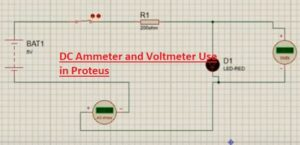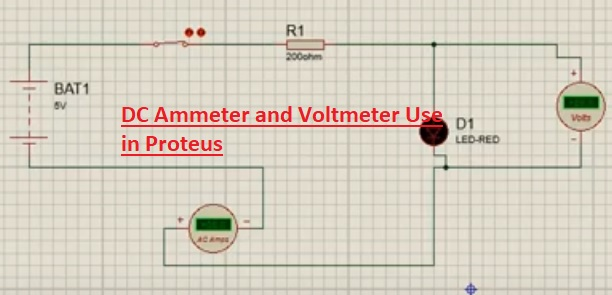Hello, readers welcome to a new post in this post we will learn How to use dc voltmeter and ammeter in Proteus. Ammeter is a current measuring device that is used in electrical circuits for measuring the current flowing in the circuit. It is generally connected in series in the current path and measures the current flowing.

A voltmeter is a voltage measuring device that is used in different circuits. It is linked in parallel combination to the circuit for volt measuring. In this post, we will learn how we can use these two devices in the proteus for measuring the current and volts of a circuit. So let get started.

## What is Ammeter

• The device that measured the current passing in the circuitry is called an ammeter.
• The resistance offered by the ammeter is less than the voltmeter.
• It should link to supply since it has less value of resistance and can be damaged due to large current flow.
• Some meter has a range of a few mA of currents.
• To increase, the measuring range of the meter there should be resistance called shunt show connected to ammeter ins parallel combination.
• It normally has a feature to operates for less voltage range normally single volts or less
• The circuit for which the current is measured ammeter is attached in a series combination.
• Its accuracy is larger than the voltmeter.
• Its range can not be varied.## What is Voltmeter

• The instrument used to find the value of voltage about two terminals of circuitry is called a voltmeter.
• The value of resistance offered by the voltmeter is larger than the ammeter.
• Its resistance value is large than the ammeter.
• The Analog meter has a needle that indicates the voltage value that to be calculated.
• To measure the small value of voltage it is linked to amplifier circuitry.
• Digital meter indicates voltage in numbers
• The circuit about which voltage is measured voltmeter is connected in parallel combination.
• It is less accurate than the ammeter.
• Its measuring range can be varied.
• Some meter gets power from an outer source and some have its own power source to operate.
• With transformer different voltmeters are used to show the value of volts at input and output.
• For measuring the volts value from some volts to thousand volts general-purpose voltmeter used
• Digital meter has a high level of correctness normally one percent than other

### DC Ammeter and Voltmeter Use in Proteus

• To learn the practical use of voltmeter and ammeter we will make a cirucit and use voltmeter and ammeter the component sof circuits are listed here.
• Battery
• Resistance
• LED
• Ammter and VOltmeter
• All components are selected from the library of proteus make the circuit as shown below figure and connected the ammeter and voltmeter to the circuit and find the current volts of the circuit as shown below